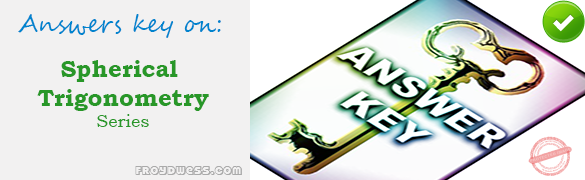# MCQ in Analytic Geometry: Points, Lines and Circles Part 2 – Answers

(Last Updated On: January 3, 2021)Below are the answers key for the Multiple Choice Questions in Analytic Geometry: Points, Lines and Circles Part 2.

51. B. IV

52. C. IV

53. C. (11, -20)

54. C. (6, -6)

55. B. (1, 1)

56. C. 5

57. A. (0, 1)

58. C. 38

59. D. 9.487

60. D. 10

61. C. 19 or –5

62. B. √2

63. B. 5.4

64. B. 2.36

65. C. 3

66. B. 3.54

67. D. (64, -44) and (4, -4)

68. D. (x/a) + (y/b) = 1

69. A. 1

70. B. -3/2

71. B. –3

72. A. 2

73. D. 77.47°

74. C. 3x – 4y – 5 = 0

75. A. 4y = 1

76. A. 3x – 2y + 1 = 0

77. D. 3x – 2y – 1 = 0

78. B. 45°

79. B. Are coincident

80. B. 5x – 4y – 1 = 0

81. C. y = 2/3 x + 1

82. C. y – x – 4 = 0

83. C. 4

84. C. 3x – 2y = 12

85. D. 2.5

86. A. 6π

87. B. 225π

88. C. 5.81

89. A. 13.86

90. B. 25π

91. A. (√33)/4

92. B. 5

93. D. 8/3

94. A. (3, -2)

95. C. x2 + y2 – 8x – 10y + 25 = 0

96. A. x2 + y2 + 4x – 6y – 12 = 0

97. B. 4x2 + 4y2 – 36x + 16y – 192 = 0

98. C. x2 + y2 + 6x – 14y + 49 = 0

99. A. x2 + y2 + 2x – 2y – 23 = 0

100. B. -5

### Online Questions and Answers in Analytic Geometry: Points, Lines and Circles Series

Following is the list of practice exam test questions s in this brand new series:

MCQ in Analytic Geometry: Points, Lines and Circles
PART 1: MCQ from Number 1 – 50                             Answer key: PART 1
PART 2: MCQ from Number 51 – 100                        Answer key: PART 2

Please do Subscribe on YouTube!

P inoyBIX educates thousands of reviewers and students a day in preparation for their board examinations. Also provides professionals with materials for their lectures and practice exams. Help me go forward with the same spirit.

“Will you subscribe today via YOUTUBE?”

Subscribe